## MP Board Class 12th Chemistry Important Questions Chapter 2 Solutions

### Solutions Important Questions

Question 1.
Write formula of van’t Hoff factor ‘i’
van’t Hoff factor, i = $$\frac {Observed value of colligative property}{Calculated value of colligative property}$$

Question 2.
Write down van’t Hoff equation. Give formula used for calculating molecular mass with its help.
van’t Hoff equation, πV = nRT
or π = $$\frac { nRT}{V}$$
or π = $$\frac { WRT}{MV}$$
or π = $$\frac { WRT}{πV}$$
Where, W is mass of solute, R is Solution constant, T is temperature, n is osmotic pressure and V is volume of Solution.

Question 3.
Define Normality.
Number of gram equivalent of solute present in one litre of Solution: is called normality. It is represented by N.
Normality (N) = $$\frac {Number of gram equivalent of solute}{Volume of Solution: in litres}$$
or N = $$\frac {Mass of solute in grams }{Equivalent mass of solute}$$ x $$\frac { 1000}{ Volume of Solution: in ml }$$
∵ [No. of gram equivalent =$$\frac {Mass of solute in grams}{Equivalent mass of solute}$$]
Normality of a Solution changes with temperature as it is based on mass – volume relationship and volume changes with change in temperature.Question 4.
Differentiate between Molarity and Molality.
Differences between Molarity and Molality :
Molarity (M):

• Molarity involves the total volume of Solution.
• In molarity, gram moles of solute are dissolved in 1 litre of Solution:.
• Molarity changes with temperature because volume changes with temperature.

Molality (m):

• Molality involves the mass of solvent.
• In molality, gram moles of solute are dissolved in 1 kg of solvent. Here volume of Solution is not considered.
• Molality is independent of temperature as it takes mass into consideration.

Question 5.
Explain the following term : Parts per million.
Parts per million:
When a solute is present in very minute amounts (in traces), the concentration is expressed in parts per million abbreviated as ppm. The parts may be of mass or volume. It is the parts of a component per million parts of the Solution.
i.e., ppm = $$\frac {Mass of component A}{Total mass of Solution:}$$ x 106
or ppm = $$\frac {Volume of component A}{Total volume of Solution:}$$ x 106
Where, ppm. is the concentration of component A in parts per million.

Question 6.
What role does the molecular interaction play in a Solution: of alcohol and water? (NCERT)
Alcohols dissolve in water due to formation of inter – molecular H – bonding with water.

Question 7.
Why do gases always tend to be less soluble in liquids as the temperature is raised? (NCERT)
Gas + Liquid ⇌ Dissolved gas   ∆ H = – ve
The dissolution of a gas in a liquid is exothermic process. Therefore in accordance with Le – Chatelier’s principle with increase in temperature, the equilibrium shifts in back-ward direction. Therefore, the solubility of gas in Solution decreases with the rise in temperature.

Question 8.
State Henry’s. (NCERT)
Henry’s law:
According to this law, ‘The mass of a gas dissolved per unit volume of a solvent at constant temperature, is proportional to the pressure of the gas with which the solvent is in equilibrium’. Let in unit volume of solvent, mass of the gas dissolved is m and equilibrium pressure be P, then m ∝P or m = KP, where K is a constant.

Question 9.
What are colligative properties?
The physical properties of Solution which depend on the number of total particles present in Solution and the ratio of number of particles of solute not on the nature of solute particles are known as colligative properties.

Question 10.
Give an example of Solution: of solid in solid.
Mixture of copper and gold is an example of solid in solid.

Question 11.
On dissolving ethanoic acid in benzene, experimental molecular mass of ethanoic acid is generally found to be double. Why?
On dissolving ethanoic acid in benzene, it dimerizes due to the formation of hydrogen bond. Thus, its experimental value is generally found to be double.

Question 12.
What is vapour pressure? What is the effect of temperature on it?
Pressure exerted by the vapours on the surface of a liquid at the state of equilibrium is known as vapour pressure. On increasing the temperature vapour pressure increases.

Question 13.
What type of deviation is represented by acetone and CS2 Solution?
Positive deviation.Question 14.
Why is CaCl2 used for clearing off snow from the roads?
On adding CaCl2, freezing point of water decreases. Therefore, CaCl2 is used for clearing off snow from the roads.

Question 15.
Based on solute – solvent interactions, arrange the following in order of increasing solubility in n – octane and explain :
Cyclohexane, KCl, CH3OH, CH3CN.
For solubility we know Tike dissolves like’, n – octane is a non – polar solvent, hence non-polar compounds will be more soluble.
KCl < CH3OH < CH3CN < Cyclohexane.

Question 16.
What is transition temperature?
The temperature at which the nature of solubility changes (i.e., first it increases, then decreases) is known as transition temperature. Solubility of sodium sulphate in water increases upto 32.4, then it starts decreasing. Thus, 32.4°C is the transition temperature of sodium sulphate.

Question 17.
Define molarity and molality. (NCERT)
Molarity “is defined as number pf gram mole§ of solute dissolved in a litre of Solution. It is denoted by M.
Molality (M) = $$\frac {Mass of solute in gram per litre}{ Molecular mass of solute}$$

Molality is defined as number of moles of solute present in a kilogram (1000 gram) of solvent. It is denoted by m.
Molality (m) = $$\frac {Mass of solute in kg of solvent}{Gram molecular mass of solute}$$

Question 1.
Write down Raoult’s law.
The vapour pressure of a solution containing non – volatile solute is directly pro – portional to the mole fraction of the solute.
Mathematically,
$$\frac { { X }_{ A } }{ { X }_{ A }+{ X }_{ B } }$$ = $$\frac { P{ A }^{ 0 } }{ { P }_{ A }+{ P }_{ B } }$$
Where, P0A = Vapour pressure of pure solvent, PA = Vapour pressure of solvent in solution, XB = Mole fraction of solute.

Question 2.
What is Azeotropic mixture? They are of how many types? (MP 2018)
Azeotropic mixture is the mixture of liquids which boil at one temperature with-out any change in composition. For example, at the composition of 95 6% alcohol and 4.4% water. It form an azeotropic mixture which boils at 78.13°C. Components of this mixture cannot be separated fully by fractional distillation.

They are of two types :
1. Low boiling azeotropic mixture:
Such Solutions which represent positive deviation towards Raoulfs law i.e. their vapour pressure is high thus their boiling point is low are known as low boiling azeotropic mixture.

Example:

• CS2 + Acetone
• C2 H5 OH + n – hexane.

2. High boiling azeotropic mixture:
Such Solutions which represent negative deviation towards Raoult’s law i.e. their vapour pressure is low thus their boiling point is high are known as high boiling azeotropic mixture.

Example:

• Acetone + Chloroform
• Ether + Chloroform.

Question 3.
What are ideal and non – ideal Solutions? Explain with example.
Ideal Solutions:
Ideal Solutions are those Solutions in which Raoult’s law can be applied completely for all concentrations of the Solutions and at all temperatures.

Condition for ideal Solutions are following :

1. PA = PA0 X A and PB= P0B X B
2. ∆Vmixing = 0 and
3. ∆Hmixing = 0 and

Example:
C2H5Br + C2H5Cl, C6H6 + C6H5CH3, CCl4 + SiCl4etc.

Non – ideal Solutions:
Solutions in which Raoult’s law cannot be applied completely for all concentrations and temperatures are called non – ideal Solutions.
For these Solutions:

1. PA ≠ P0A XA and pB ≠ P0BX
2. ∆Vmixing ≠ 0 and
3. ∆Hmixing ≠ 0 and

Example:
Benzene + Acetone, CHCl3 + HNO3 etc.Question 4.
Establish van’t Hoff Solution: equation. (MP 2018)
Osmotic pressure of dilute Solution of a non – volatile solute is proportional to absolute temperature of the Solution at constant concentration. This is known as van’t Hoff law.
π ∝ T .. (1)
Derivation : Osmotic pressure n of a Solution: is directly proportional to a molar concentration.
π ∝ C
By eqn.(l) and eqn.(2) π ∝ C (T is constant) .. (2)
or π = RCT .. (3)
Where, R = Gas constant
or
C = $$\frac {1}{V}$$
π = $$\frac {RT}{V}$$ .. (4)
πV = RT .. (5)
This is known as van’t Hoff Ideal Solution equation.

Question 5.
Define the following:

1. Molal elevation boiling point constant.
2. Molal freezing point depression constant. (MP 2018)

1. Molal elevation boiling point constant : Molal elevation constant can be defined as “The elevation in boiling point of the Solution in which 1 gm of solute is dissolved in 1000 gm of solvent.”
∴ Elevation in boiling point
∆ Tb ∝ m
∆ Tb = Kbm
m= 1, ∆ Tb = Kb
Where Kb = Molal boiling point elevation constant.

2. Molal freezing point depression constant : Molal depression constant may be defined as “The depression in freezing point for 1 molal Solution: i.e., Solution: in which 1gm mole of solute is dissolved in 1000 gm of solvent.”
∴ Depression in freezing point
∆ Tf ∝ m
∆ Tf = kf
If m = 1, ∆ Tf = kf
Where Kf = Molal freezing point depression constant.

Question 6.
What is Raoult’s law? Establish its mathematical expression.
Or,
What is Raoult’s law? How can molar mass of a non – volatile solute be determined with its help?
Raoult’s law:
For a Solution in which solute is non – volatile, the Raoulf s law may be stated as following :
“At any constant temperature, vapour pressure of solvent collected above the Solution of non – volatile solute, is directly proportional to the mole fraction of solute.” If a non – volatile solute is added to a volatile solvent, the vapour pressure of the solvent decreases. The vapour pressure of the solvent is directly proportional to its mole fraction. As the solute is non – volatile, the vapour pressure of the Solution (P) will be equal to the vapour pressure of the solvent (PA).
P = PA ∝ XA
or PA=KXA .. (1)
Where, K = Proportionality constant.
Apply eqn. (1) for pure solvent if XA= 1 and PA = P0A
Then, P0A = K x 1 .. (2)
Where, p0A= Vapour pressure of pure solvent.
Putting the value of K from eqn. (2) in eqn. (1),
PA=P0AXA .. (3)
Where, XA is the mole fraction of the solvent in the Solution.
The mole fraction of solute is represented as XB.
So, XA+XB=1
or XA= 1 – XB .. (4)
From eqns. (3) and (4),
PA= P0A(1-XB) = P0 – P0AXB
XB = $$\frac { { P }_{ A\quad }^{ 0 }-\quad { P }_{ A } }{ { P }_{ A }^{ 0 } }$$
P0A is lowering in vapour pressure and $$\frac { { P }_{ A\quad }^{ 0 }-\quad { P }_{ A } }{ { P }_{ A }^{ 0 } }$$ is relative lowering in vapour pressure.

On the basis of equation (5) Raoult’s law can be defined as “The relative lowering in vapour pressure of a Solution containing non – volatile solute is equal to mole fraction of solute”.Question 7.
Give relation between elevation in boiling point and molecular mass of solute.
Relation between elevation in boiling point and molecular mass of solute:
Suppose, WB gram of non – volatile solute dissolve in WA gram of solvent and the molecular mass of non – volatile solute is MB gram. Then, molality, m will be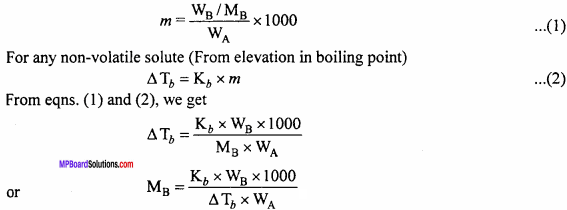Question 8.
What is van’t Hoff factor? How does it equation?
van’t Hoff Equation : van’t Hoff equation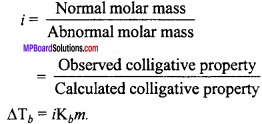Question 9.
What are constant boiling mixture? Write three differences in Ideal solution and Non – ideal Solutions. (MP 2011,15,16)
Constant boiling mixtures or azeotropic mixture. A Solution which distils with out change in composition is called azeotropic mixture.
Differences between Ideal and Non – ideal Solution: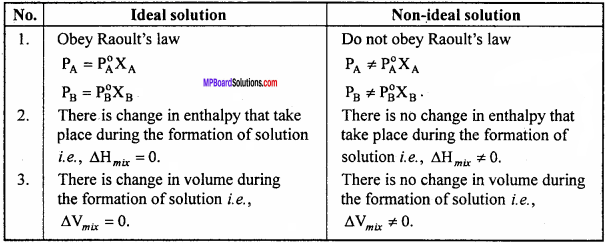Question 10.
Define the following :

1. Reverse osmosis
2. Isotonic Solution
3. Semi – permeable membrane.

1. Reverse osmosis:
It may be noted that if a pressure higher than the osmotic pressure is applied on the Solution the solvent will flow from the Solution into the pure solvent through the SPM. The process is called reverse osmosis.

2. Isotonic Solutions:
The Solution:s which have same osmotic pressure are called as isotonic Solution:s. For example, 0.91% Solution of NaCl called as saline water is isotonic with human blood corpuscles. That is why medicines are mixed with saline water before intravenous injections.

Osmotic pressure is a colligative property and depends on the number of solute particles in a Solution So, the isotonic Solution:s must have same number of solute particles in a given volume of Solution Consequently, the isotonic Solution:s are those which have same molality, if that the solute neither associate nor dissociate in the Solution.

3. Semipermeable membrane:
These are the membrane which allow the movement of the solvent molecules through them. The membrane appear to be continuous sheets or films. But they have very tiny holes or pores which are semimicro in nature. Through the holes, only the molecules of solvent can pass while those of bigger solute molecules cannot pass through.

Question 1.
Write five differences in Solution: having Positive deviation and Negative deviation.
Differences between Positive deviation and Negative deviation :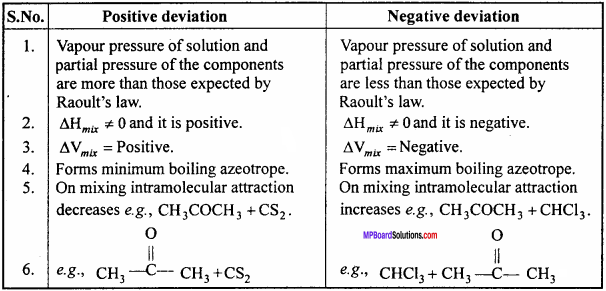Question 2.
Explain in brief Berkeley and Hartley’s method of osmotic pressure measurement and state its uses.
Berkeley and Hartley’s method:
In this method, pressure is applied over the Solution to stop the flow of solvent. This pressure is equivalent to osmotic pressure.
In this method, the apparatus consists of a strong vessel made up of steel in which porous pot is fitted. In the porous pot, copper ferrocyanide semipermeable membrane is deposited. The porous pot is fitted with a capillary tube on one side and a water reservoir on the other side. A piston and pressure gauge are fitted to the steel vessel.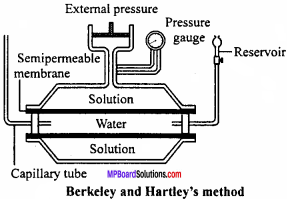The porous pot and steel vessel are filled with water and Solution respectively. Osmosis takes place and water moves into the steel vessel from the porous pot through the semipermeable membrane. This is shown by fall in water level in the capillary tube. This flow of water is stopped by applying external pressure on the Solution with the help of piston.

This method has the following advantages :

1. It takes comparatively lesser time to determine osmotic pressure.
2. Concentration of Solution: does not change, hence better results are obtained.
3. As high pressure is not exerted over semipermeable membrane, it does not break.
4. High osmotic pressure can be measured.

Question 3.
What is molal freezing point depression constant? Derive the formula to establish relation between molal freezing point depression constant and molecular mass of solute.
Or,
What is molal freezing point depression constant? Show that depression in freezing point is a colligative property. How can molecular mass of solute be determined from depression in freezing point?
Molal freezing point depression constant is equal to depression in freezing point of the Solution when 1 gm mole is dissolved in 1000 gm of solvent. It is represented by i.e.,
Kf = $$\frac {depression in freezing point}{Number of moles}$$
or ∆ Tf = Kf x m
As Kf is molal freezing point depression constant, then
∆ Tf ∝m
Where, m = Number of moles dissolved in 1000 gm solvent.
Thus, depression in freezing point is proportional to molality of Solution. Molality is directly proportional to number or molecules of solute substance. Therefore, depression in freezing point is a colligative property.
Calculation of molecular mass of solute:
By determination of depression in freezing point, the Molecular mass of non – volatile solute can be determined.
For a Solution: of non – volatile solute,
∆Tf = Kf x m .. (1)
Let WB gram non – volatile solute is dissolved in wA gram solvent and molecular mass of solute is MB.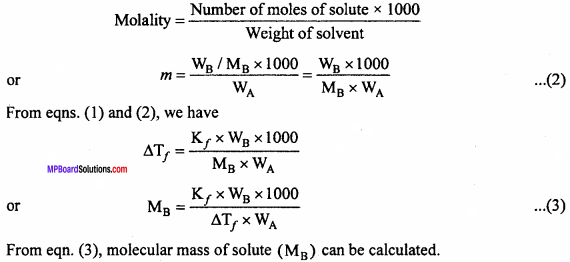Question 4.
What is elevation in boiling points? How addition of a non – volatile solute elevates the boiling point of a solvent? Explain it with the help of graph diagram.
The vapour pressure of the Solution:
containing a non – volatile solute is always less than that of pure solvent. Therefore, the Solution has to be heated to higher temperature so that its vapour pressure become equal to the atmospheric pressure. Thus, the boiling point of Solution (Tb)is always higher than the boiling point of solvent (Tb0). The difference Tb – Tb0 is called elevation in boiling point.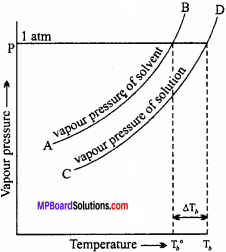If we plot graph between temperature and vapour pressure of a pure solvent and its Solution, then following curve is obtained. Curve AB gives the vapour pressure for the pure solvent and the curve CD gives the vapour pressure of the Solution: at different temperature. At temperature Tb0 the vapour pressure of the solvent becomes equal to the atmospheric pressure hence it boils at Tb0.

Now, by the addition of non – volatile solute, lowering of vapour pressure of the Solution takes place. And to increase the vapour pressure of the Solution to become equal to atmospheric pressure, the temperature rises. Hence, at Tb the Solution boils. Thus, the boiling point is now elevated from Tb0 to Tb. The rise in temperature that results by the addition of a non – volatile solute in a solvent is termed as elevation in boiling point. It is represented by ∆Tb.
So, elevation in boiling point (∆Tb) = Tb – Tb0.

Question 5.
What are Non – Ideal Solution? How many types of are they? Explain with giving examples.
Non – ideal Solutions:
Solutions in which Raoult’s law cannot be applied completely for all concentrations and temperatures are called non – ideal Solutions.
For these Solutions:

1. PA ≠ P0A XA and pB ≠ P0BXB,
2. ∆Vmixmg ≠ 0 and
3. ∆Hmixmg ≠ 0 and

Non – ideal Solution are of two types :

1. Solutions showing positive deviations:
For such Solutions the total vapour pressure will be greater than the corresponding vapour pressure according to the Raoult’s law. The boiling point of such Solutions are lowered. Because interactions between the mol-ecules of Solution: is less than the interactions between pure solvents and solutes. Thus the vapour pressure greater than expected. In these type of Solution formed enthalpy and volume increases.
Characteristics:

• PA > P0A XA and PB > P0B XB
• ∆Vmixlng > 0
• ∆Hmixlng > 0

Example:
Solution of Cyclohexane and Ethanol:
In Ethanol, its molecules are held together by hydrogen bond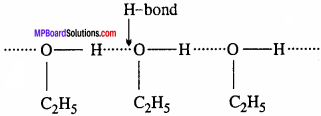On adding cyclohexane, the molecules tend to occupy the space between ethyl alcohol molecules. As a result the attractive forces between alcohol molecules becomes less. Thus, formation of such Solution: is slight increase in vapour pressure, endothermic and an increase in volume.

2. Solutions showing negative deviations:
For such Solutions the total vapour pressure becomes less than expected according to the Raoult’s law. Because interaction between molecules of Solution is greater than interaction between the pure solvent or solute molecules. Thus the vapour pressure of Solution is less. In these type of Solutions formed enthalpy and volume decreases.
Characteristics:

• PA < P0A XA and PB < P0B XB
• ∆Vmixlng < 0
• ∆Hmixlng < 0

Example:
Solution of Acetone and Chloroform:
When acetone and chloroform are mixed, there are new attractive forces due to intermolecular hydrogen bonding. These force become stronger. Thus the formation of such Solution:s is an exothermic and the vapour pressure of Solution is less and decrease in volume.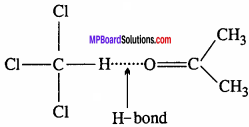Solutions Numerical Questions

Question 1.
Calculate the mass of urea (NH2 CONH2 ) required in making 2.5 kg of 0.25 molal aqueous Solution. (NCERT)
Solution:
0.25 molal aqueous Solution means that
Moles of urea = 0.25 mole
Mass of solvent (water) = 1 kg = 1000 g
Molar mass of urea = 14+ 2 +12+ 16 +14+ 2 = 60 g mol-1
∴ 0 .25 mole of urea = 60 x 0.25 mole = 15 g
Total mass of the Solution = 1000 + 15 g
= 1015 g = 1.015 g
∵ 1.015 kg of Solution contain urea = 15 g
∴ 2.5 kg of Solution will require urea = $$\frac {1.5}{1.015}$$ x 2.5 kg = 37 g.

Question 2.
Henry’s law constant for CO2 in water is 1.67 x 108 Pa at 298 K. Calculate the quantity of CO2 in 500 ml of soda water when packed under 2.5 atm CO2 pressure at 298 K. (NCERT)
Solution:
According to Henry’s law,
P = KHX .. (1)
P = 2.5 atm = 2.5 x 101325 Pa, KH= 1.67 x 108 Pa
Putting these values in equation (1), we get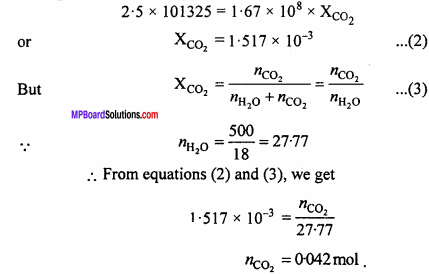Question 3.
Calculate the mass of ascorbic acid (Vitamin C, C6H8O6) to be dissolved in 75 g of acetic acid to lower its melting point by 1.5°C. KfK kg mol-1. (NCERT)
Solution:
We know that ∆Tf = Kf x $$\frac{\mathrm{W}_{\mathrm{B}} \times 1000}{\mathrm{M}_{\mathrm{B}} \times \mathrm{W}_{\mathrm{A}}}$$ .. (1)
Given ∆Tf = 1.5, Kf = 3.9 K kg mol-1, WA = 75g, MB (ascorbic acid, C6H8O6)
= 6 x 12 + 8 x l + 16 x 6 = 176
Putting, these values in equation (1),
1.5 = 3.9 x $$\frac{\mathrm{W}_{\mathrm{B}} \times 1000}{176 \times 75}$$
WB = 5.077g.Question 4.
Calculate the osmotic pressure in pascals exerted by a Solution prepared by dissolving 1.0 g of polymer of molar mass 1,85,000 in 450 ml of water at 37°C. (NCERT)
Solution:
π = CRT
= $$\frac {n}{V}$$ RT
Here, number of moles of solute dissolved (n)
= $$\frac {1.0}{185,000}$$ mol-1
= $$\frac {1}{185,000}$$ g mol-1
v = 450mL = 0.450 L
T = 37°C = 37 + 273 = 310 K
R = 8.314 K Pa LK-1 mol-1
= 8.314 x 103 Pa LK-1 mol-1
Substituting these values we get,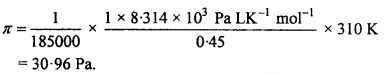Question 5.
Concentrated nitric acid used in laboratory work is 68% nitric acid by mass in aqueous Solution. What should be the molarity of such a sample of the acid if the density of the Solution is 1.504 g ml-1? (NCERT)
Solution:
68% nitric acid by mass means that
Mass of nitric acid = 68 g
Mass of Solution = 100 g
Molar mass of HNO3 = 63 g mol-1
∴ 68 g HNO3 = $$\frac {68}{63}$$ mole = 1.079 mole
Density of solution = 1.504 g mL-1
∴ Volume of solution = $$\frac {100}{1.504}$$mL = 66.5 mL = 0 0665 L
Molarity of the solution = $$\frac {Moles of the solute}{Volume of Solution in L}$$
= $$\frac {1.079}{0.0665}$$ M = 16.23 M.

Question 6.
Vapour pressure of water at 293 K is 17.535 mm Hg. Calculate the vapour pressure of water at 293K when 25g of glucose is dissolved in 450g of water. (NCERT)
Solution:
$$\frac { { p }^{ 0 }-{ p }^{ 0 } }{ { p }^{ 0 } }$$ = X2 = $$\frac { { W }_{ B }{ M }_{ A } }{ { M }_{ B }{ W }_{ A } }$$
or $$\frac { 17.535-{ P }_{ s } }{ 17.535 }$$ = $$\frac { 25×18 }{ 180×450 }$$
or $$\frac { 17.535-{ P }_{ s } }{ 17.535 }$$ = 5.56×10-3
17.535 – ps = 0.0975
Ps = 17.438 mm Hg.

Question 7.
Henry’s law constant for the molality of methane in benzene at 298 K is 4.27 x 105mm Hg. Calculate the solubility of methane in benzene at 298K under 760 mm Hg. (NCERT)
Solution:
Here, KH = 4.27 x 105 mm, P = 760 mm
Applying Hemy’s law, P = KHX
$$\mathrm{X}=\frac{\mathrm{P}}{\mathrm{K}_{\mathrm{H}}}=\frac{760}{4 \cdot 27 \times 10^{5}}$$ =1.78 x 10-3
∴ Mole fraction of methane in benzene = 1.78 x 10-3Question 8.
Benzene and toluene form ideal solution over the entire range of composition. The vapour pressure of pure benzene and toluene at 300K are 50.71 mm Hg and 32.06 mm Hg respectively. Calculate the mole fraction of benzene in vapour phase if 80 g of benzene is mixed with 100 g of toluene. (NCERT)
Solution:
A → Benzene (C6H6); B —> Toluene (C7H8)
Number of moles of benzene nA = $$\frac {80}{78}$$
Number of moles of toluene = nB = $$\frac {100}{92}$$
XA = $$\frac { { n }_{ A } }{ { n }_{ A }+{ n }_{ B } } \quad$$ = $$\frac {1.026}{1.026+1.087}$$ = 0.486
XB = 1 – XA = 1 – 0.486 = 0.514
Given P°A = 50.71 mm Hg and P°B = 32.06 mm Hg
We know, P = PA + PB
= P°AXA + P°AXB
= (50.71 x 0.486) + (32.06 x 0.514)
= 24.65+ 16.48 = 41-13
Mole fraction of components in vapour phase may be calculated by using Dalton’s law,
PA = yA x P
yA = $$\frac { { P }_{ A } }{ p } \quad$$ = $$\frac {24.65}{41.13}$$ = 0.60

Question 9.
Determine the osmotic pressure of 5% glucose Solution at 25°C. Molecular mass of glucose = 180, R = 0.0821 litre atmosphere. (MP 2013,16)
Solution:
∴5 gm glucose is dissolved in 100 ml.
∴ 180 gm glucose will be dissolved in $$\frac {100}{5}$$ x 180
= 3600 ml = 3.6 litre.
We know that,
PV = RT
P x 3.6 = 0.0821 x (25 + 273) = 298
or P = $$\frac {0.52×12.5×1000}{0.63×170}$$
= 6.80 atmospheres.Question 10.
12.5 gm of urea dissolved in 170 gm of water. The elevation in boiling point was found to be 0.63 K. If KA for water = 0.52 Km1, calculate the molecular mass of urea. (MP 2010)
Solution:
Formula: MB$$\frac { { K }_{ b }x{ W }_{ B }x1000 }{ ∆{ T }_{ b }x{ W }_{ A } }$$
Given: WA = 170gm,WB= 12.5gm, ∆TB=0.63K, Kb=0.52Km-1
MB = $$\frac {0.52×12.5×1000}{0.63×170}$$
MB = 60.7 gm mol-1

Question 11.
If 6.84 gram sucrose is dissolved in 100 ml. Solution then what will be its osmotic pressure at 20°C? (R = 0.082 litre atmosphere K-1mol-1) (Molecular mass of sucrose MB = 342)
Solution:
Given : Weight of solute (WB) = 6.84 gm
Molecular mass of solute (MB) = 342
Volume of Solution: (V) =$$\frac {100}{1000}$$ =0.1 litre
Temperature (T) = 20° C = 273 + 20 = 293 K
Solution constant R = 0.082 litre atmosphere K-1 mol-1
Osmotic pressure π = $$\frac { { W }_{ B }RT }{ { M }_{ B }V }$$
= $$\frac {6.84×0.082×293}{342×0.1}$$
= 4.8 atmosphere.